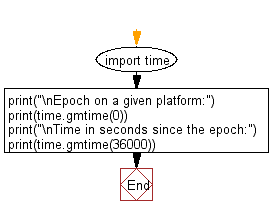﻿ Python: Find out what the epoch is on a given platform - w3resource# Python: Find out what the epoch is on a given platform

## Python Datetime: Exercise-55 with Solution

The epoch is the point where the time starts, and is platform dependent. For Unix, the epoch is January 1, 1970, 00:00:00 (UTC). Write a Python program to find out what the epoch is on a given platform. Also convert a given time in seconds since the epoch.

Sample Solution:

Python Code:

``````import time
print("\nEpoch on a given platform:")
print(time.gmtime(0))
print("\nTime in seconds since the epoch:")
print(time.gmtime(36000))
```
```

Sample Output:

```Epoch on a given platform:
time.struct_time(tm_year=1970, tm_mon=1, tm_mday=1, tm_hour=0, tm_min=0, tm_sec=0, tm_wday=3, tm_yday=1, tm_isdst=0)

Time in seconds since the epoch:
time.struct_time(tm_year=1970, tm_mon=1, tm_mday=1, tm_hour=10, tm_min=0, tm_sec=0, tm_wday=3, tm_yday=1, tm_isdst=0)
```

Flowchart:## Visualize Python code execution:

The following tool visualize what the computer is doing step-by-step as it executes the said program:

Python Code Editor:

What is the difficulty level of this exercise?

Test your Python skills with w3resource's quiz

﻿

## Python: Tips of the Day

Free up Memory:

• Manual garbage collection can be performed on timely or event based mechanism.
```import gc
collected_objects = gc.collect()
```